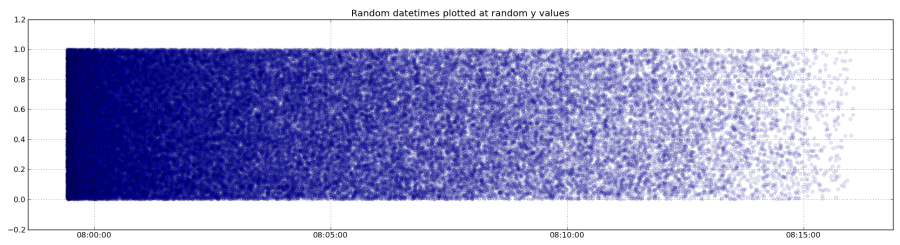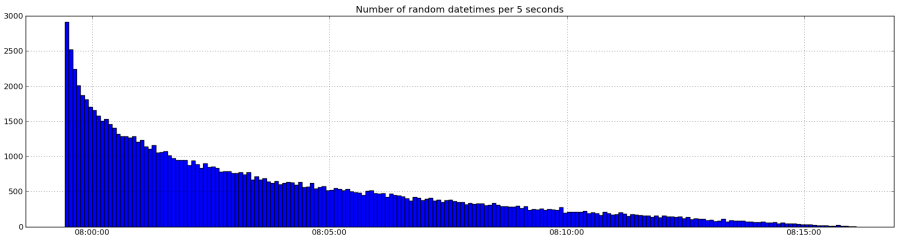# Matplotlib & Datetimes – Tutorial 03: Grouping Sparse Data

New tutorial, more advanced this time ! Let’s say we have a number of observations, like occurrences of earthquakes, or visitors connecting to a webserver, etc. These observations don’t occur every second, they are sparse on the time axis. To prepare an example, I’ve created a set of random datetimes like this :

```N = 100000
starttime = time.time()
basetimes = sorted(np.random.random(N)*np.random.random(N)*1.0e3+starttime)
times = [datetime.datetime(*time.gmtime(a)[:7]) for a in basetimes]
for i, atime in enumerate(times):
times[i] = atime + datetime.timedelta(microseconds=(basetimes[i]-int(basetimes[i])) * 1e6)```

N being the number of points we want. We also need to loop over the original times array to add the microseconds, because time.gmtime doesn’t take them into account. This times array can be plotted using:

```fig = plt.figure()

ax = plt.subplot(211)
plt.scatter(times,np.random.random(len(times)),alpha=0.1)
ax.xaxis_date()
plt.grid(True)
plt.title('Random datetimes plotted at random y values')```

Note we add some random values for the Y axis, just to make a nice plot !So, now we have an array of datetime.datetime objects and we want to get the number of occurrences on a certain time span, let’s say, 5 seconds. First, to work easily, we convert the array to a numpy array. The binning will be done using the powerful itertools.groupby method. It takes two arguments: an iterable, here our times array, and a key, which can be the name of a method.

itertools.groupby(iterable[, key])
the key in our case will be a method that will return the timestamp int-divided by the time span we have. So, for example, occurences at 1000.02, 1001.12, 1004.66 will get the same integer value of 200, because int(1000.02)/5=200, e.g.
```SECOND = 1
MINUTE = SECOND * 60
HOUR = MINUTE * 60
DAY = HOUR * 24

binning = 5*SECOND

def group(di):
return int(calendar.timegm(di.timetuple()))/binning

list_of_dates = np.array(times,dtype=datetime.datetime)
grouped_dates = [[datetime.datetime(*time.gmtime(d*binning)[:6]), len(list(g))] for d,g in itertools.groupby(list_of_dates, group)]
grouped_dates = zip(*grouped_dates)```
This code first shows the definition of the binning (“time-span”) and the group function that will return the key for the groupby method to group the data. The output of this method are two arrays d and g, containing the key and the elements matching each key, respectively. We then, in the long one-liner, create an array of datetime.datetime objects, multiplying back the key (=d) by the binning value to get the left corner of the bin and getting the length of the listed g array, the number of occurrences for each key. Finally, the grouped_dates array is zipped to get two arrays that will be easy to plot. The rest is super simple:
```ax = plt.subplot(212,sharex=ax)
plt.bar(grouped_dates,grouped_dates,width=float(binning)/DAY)
ax.xaxis_date()
plt.grid(True)
plt.title('Number of random datetimes per %i seconds' % binning)```
We just have to create a bar plot of the left=keys, height=”length of the group” and width= “the time span” (relative to the matplotlib.dates default unit, 1 DAY) and voilà, it’s done!As always, I’d be happy to hear about your experiments/applications of this tutorial !
The full code is after the break:
```import numpy as np
import matplotlib.pyplot as plt
import datetime, time, calendar
from matplotlib.dates import num2date, DateFormatter

import itertools

N = 100000
starttime = time.time()
basetimes = sorted(np.random.random(N)*np.random.random(N)*1.0e3+starttime)
times = [datetime.datetime(*time.gmtime(a)[:7]) for a in basetimes]
for i, atime in enumerate(times):
times[i] = atime + datetime.timedelta(microseconds=(basetimes[i]-int(basetimes[i])) * 1e6)

SECOND = 1
MINUTE = SECOND * 60
HOUR = MINUTE * 60
DAY = HOUR * 24

binning = 5*SECOND

def group(di):
return int(calendar.timegm(di.timetuple()))/binning

list_of_dates = np.array(times,dtype=datetime.datetime)
grouped_dates = [[datetime.datetime(*time.gmtime(d*binning)[:6]), len(list(g))] for d,g in itertools.groupby(list_of_dates, group)]
grouped_dates = zip(*grouped_dates)

#Let's plot !
fig = plt.figure()

ax = plt.subplot(211)
plt.scatter(times,np.random.random(len(times)),alpha=0.1)
ax.xaxis_date()
plt.grid(True)
plt.title('Random datetimes plotted at random y values')

ax = plt.subplot(212,sharex=ax)
plt.bar(grouped_dates,grouped_dates,width=float(binning)/DAY)
ax.xaxis_date()
plt.grid(True)
plt.title('Number of random datetimes per %i seconds' % binning)

plt.show()```

## 3 thoughts on “Matplotlib & Datetimes – Tutorial 03: Grouping Sparse Data”

1.Budhi says:

I have four coloum and the first coloum is data time in 5 minutes interval (e.g [09:00, 09:05,09:10… 15:00]). another coloum is another data as y values. I would like to ask question, how to make first coloum as X axis in 5 minutes interval and another coloum as y values? what library i should learn? Thank you before…